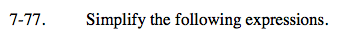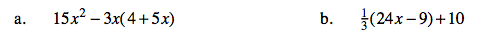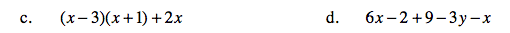### Home > AC > Chapter 7 > Lesson 7.2.3 > Problem7-77

7-77.
1. Simplify the following expressions. Homework Help ✎

1. 15x2 − 3x(4 + 5x)

2.(24x − 9) + 10

3. (x − 3)(x + 1) + 2x

4. 6x − 2 + 9 − 3yxDistribute to remove the parenthesis.
15x² − 12x − 15x²